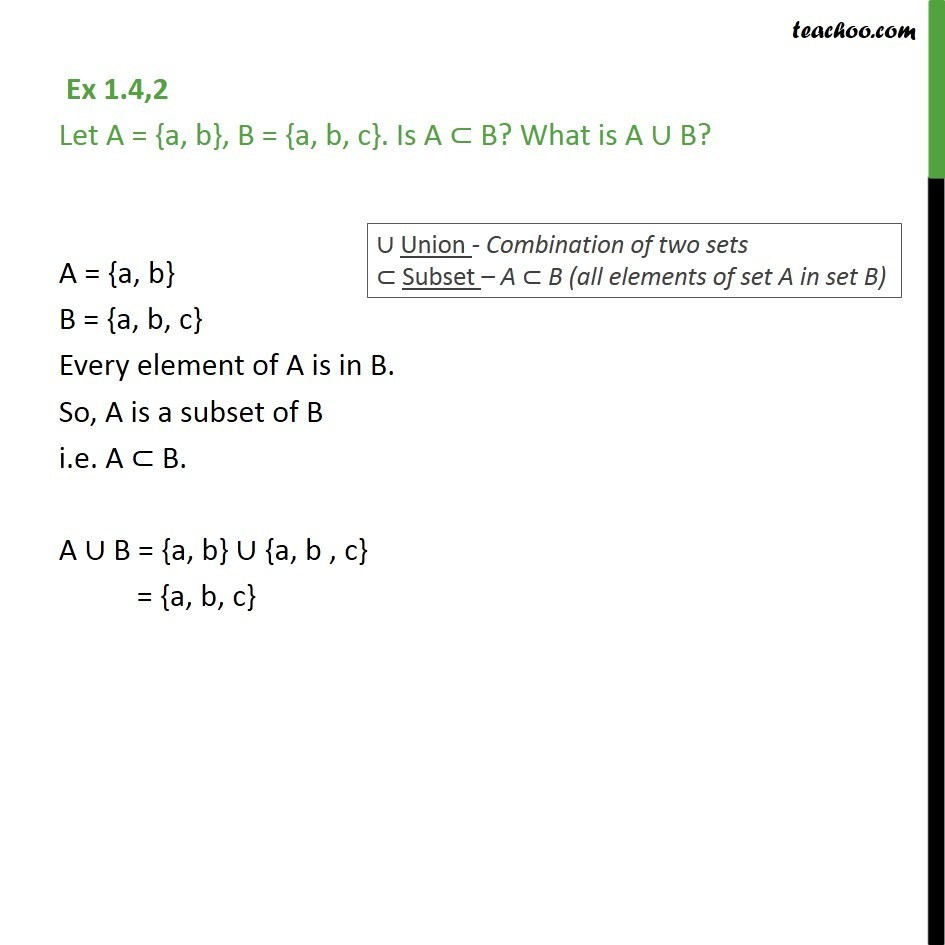1. Chapter 1 Class 11 Sets
2. Concept wise
3. Venn Diagram and Union of Set

Transcript

Ex 1.4,2 Let A = {a, b}, B = {a, b, c}. Is A B? What is A B? A = {a, b} B = {a, b, c} Every element of A is in B. So, A is a subset of B i.e. A B. A B = {a, b} {a, b , c} = {a, b, c}

Venn Diagram and Union of Set

About the AuthorDavneet Singh
Davneet Singh is a graduate from Indian Institute of Technology, Kanpur. He has been teaching from the past 9 years. He provides courses for Maths and Science at Teachoo.